Equivalent Resistance of Resistors (Parallel)
 1) When two resistances R₁ and R₂ are connected in parallel their equivalent resistance is R= (R₁R₂)/(R₁+R₂) R= (R₁+R₂)/(R₁R₂) R= (R₁R₂)/(R₁-R₂) R= (R₁-R₂)/(R₁R₂)

 2) When the three resistances are connected in parallel combination, then the effective resistance ________. decreases increases may increase or decrease depends up on the material does not change

 3) A student combines the two resistors in parallel combination, the number of common point he found is One Two Three Four

 4) Two or more resistors are said to be in parallel if one end of a resistor is connected to Other end of the other resistor One end of the other resistor One end of the voltmeter None of these

 5) Ten identical resistances each 1Ω are connected in parallel. This combination has an effective resistance of: 10 Ω 1 Ω 0.1 Ω 5 Ω

 6) A student combined n resistors, each of resistance R. The value of the smallest resistance that he can obtain by combining them is n²R R/n n/R None of these

 7) When the temperature of a metallic conductor increases, its resistance ________. always increases. always decreases. remains the same. increases first and then decreases.

 8) As length increases, what happens to the resistance? Decreases. Increases. Remains the same. May increase or decrease.9)On plugging the key, the voltmeter / ammeter is likely to be damaged in the circuit shown in which figure?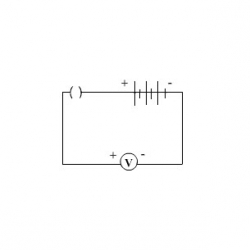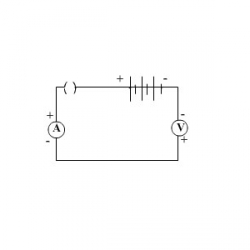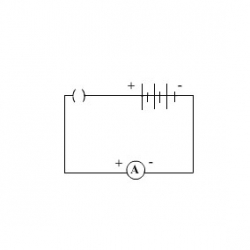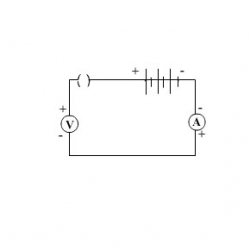10) Which of the circuit components in the following circuit diagram are connected in parallel?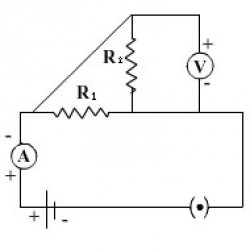R₁ and R₂ only. R₂ and V only. R₁ and V only. R₁, R₂ and V.

Cite in Scientific Research:

Nedungadi P., Raman R. & McGregor M. (2013, October). Enhanced STEM learning with Online Labs: Empirical study comparing physical labs, tablets and desktops. In Frontiers in Education Conference, 2013 IEEE (pp. 1585-1590). IEEE.

Cite this Simulator: